Author: Oscar Cronquist Article last updated on February 02, 2023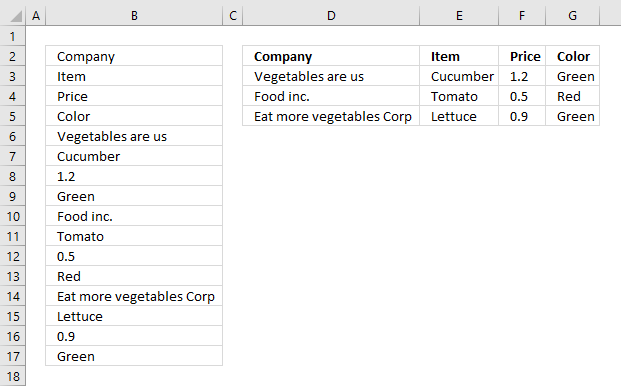The picture above shows data presented in only one column (column B), this happens sometimes when you get an undesired output from a database or copying values from an HTML file.

The formula in cell D2 extracts values from column B to a cell range containing four columns and 4 rows.

## 1. Rearrange values from a single column cell range to many columns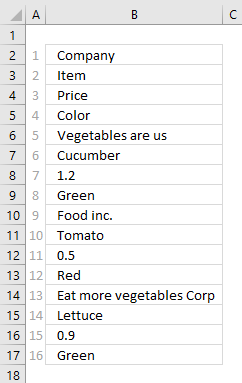The INDEX function allows you to easily rearrange values on an Excel worksheet, in this case, data seems to be grouped record by record. 4 values in one record. Column D to G shows you the INDEX function rearranging the data.

The INDEX function has three arguments: INDEX(reference, row_num, [column_num])

The reference in the first argument points to cell range B2:B17. To return a value from that cell range you must know where it is, the INDEX function uses a row and column number to locate a particular value. Since this cell range (B2:B17) only has one column you only need to use a row number to get the value you want.

The picture above has relative row numbers in column A to show you what number the INDEX function needs for it to return a specific value from cell range B2:B17. The values in column B seems to repeat, every 4th row has a company name. We can use that information to build the formula that returns a record in a row each.

The formula needs numbers in a determined sequence depending on where on the worksheet it is. If I enter the formula in cell D2 it must get values in the order shown in the picture below.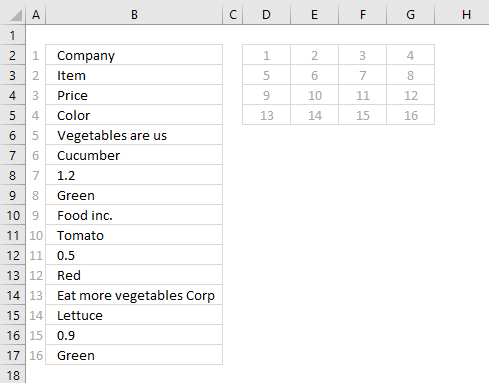Combining the ROWS function and the COLUMNS function lets you build the number sequence shown above.

Formula in cell D2:

=INDEX(\$B\$2:\$B\$17, COLUMNS(\$A\$1:A1)+ROWS(\$A\$1:A1)*4-4)

### Explaining formula in cell D2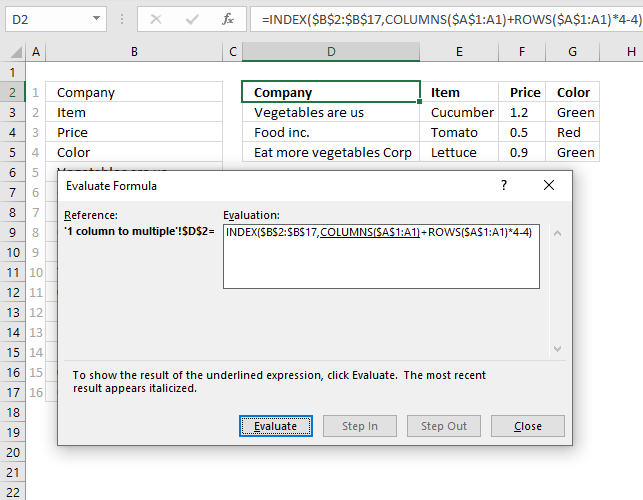I almost always use the "Evaluate Formula" tool located on the Formulas tab on the ribbon. It allows me to see each calculation step the formula performs simply by pressing a button.

The underlined expression shows what part of the formula will be evaluated when you press with left mouse button on the "Evaluate" button. The italicized expression shows the most recent result of the evaluation.

Press with left mouse button on the "Evaluate button" to see the next step being calculated, keep press with left mouse button oning the "Evaluate" button and you will reach the final result. This can take quite some time if the formula has many steps to evaluate.

#### Step 1 - Calculate the number of columns in cell range

The COLUMNS function returns the number of columns in a given cell range. Cell reference \$A\$1:A1 contains both an absolute part \$A\$1 and a relative part A1 which makes the reference expanding when the cell is copied to adjacent cells.

Note, this formula requires you to copy the cell not the formula in order to make the expanding cell reference work properly.

COLUMNS(\$A\$1:A1) returns 1.

#### Step 2 - Calculate the number of rows in cell range

The ROWS function returns the number of rows in a given cell range. Cell reference \$A\$1:A1 is also expanding when the cell is copied to adjacent cells.

ROWS(\$A\$1:A1)*4

becomes

1*4

We need to multiply the result with 4 because we want to populate four columns. Change this value if you need a cell range with more or fewer columns.

1*4 returns 4.

#### Step 3 - Add numbers and subtract with 4

COLUMNS(\$A\$1:A1)+ROWS(\$A\$1:A1)*4-4

becomes

1+4-4

We need to subtract with four to make the first row zero. The next row will then evaluate to 4 and so on.

1+4-4 returns 1.

#### Step 4 - Get values

The INDEX function gets a value from cell range or array based on a row and column number, the column number is optional if you are working with a cell range that has only one column.

INDEX(\$B\$2:\$B\$17, COLUMNS(\$A\$1:A1)+ROWS(\$A\$1:A1)*4-4)

becomes

INDEX(\$B\$2:\$B\$17, 1)

and returns the value in cell B2 which is "Company".

Here is how the cell references change when the cell is copied to adjacent cells.

In cell D2 COLUMNS(\$A\$1:A1) returns 1 and ROWS(\$A\$1:A1)*4-4 returns 0 (zero). 1 +0 is 1. The INDEX function returns the first value in cell range B2:B17 which is "Company".

In cell E2 COLUMNS(\$A\$1:A2) returns 2 and ROWS(\$A\$1:A1)*4-4 returns 0 (zero). 2+0 is 2. The INDEX function returns the second value in cell range B2:B17 which is "Item".

In cell D3 COLUMNS(\$A\$1:A1) returns 1 and ROWS(\$A\$1:A2)*4-4 returns 4. 1+4 is 5. The INDEX function returns the fifth value in cell range B2:B17 which is "Vegetables are us".## 2. Rearrange values from a single column cell range to a multicolumn cell range - Excel 365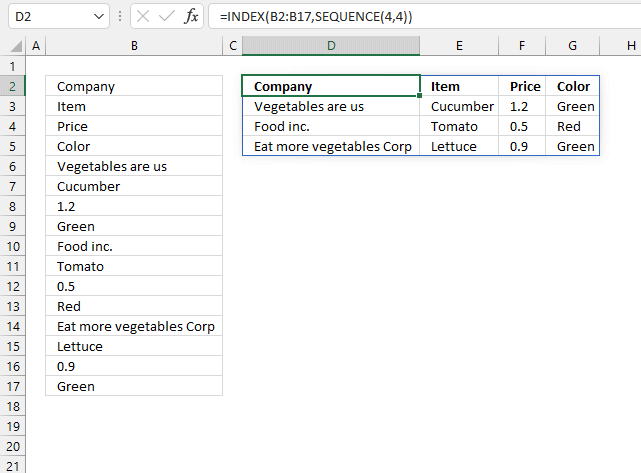This formula is a dynamic array formula that works only in Excel 365, it contains a new function namely the SEQUENCE function which can create a sequence of numbers.

Regular formula in cell D2:

=INDEX(B2:B17, SEQUENCE(4, 4))

### 2.1 Explaining formula in cell D2

#### Step 1 - Create a sequence from 1 to n

The SEQUENCE function creates a list of sequential numbers to a cell range or array.

SEQUENCE(rows, [columns], [start], [step])

The following setup returns an array of numbers from 1 to 16 split into four columns and four rows.

SEQUENCE(4,4)

returns

{1, 2, 3, 4; 5, 6, 7, 8; 9, 10, 11, 12; 13, 14, 15, 16}

The comma is a column delimiting character and the semicolon is a row delimiting character. This is based on your computer settings.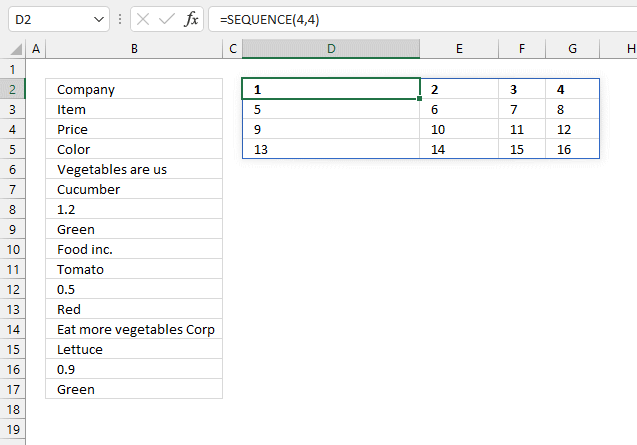#### Step 2 - Get values from cell range B2:B17

The INDEX function returns a given value from a cell range based on a row and column number. The column number is optional.

INDEX(array[row_num][column_num])

INDEX(B2:B17,SEQUENCE(4,4))

becomes

INDEX(B2:B17, {1, 2, 3, 4; 5, 6, 7, 8; 9, 10, 11, 12; 13, 14, 15, 16})

becomes

INDEX({"Company"; "Item"; "Price"; "Color"; "Vegetables are us"; "Cucumber"; 1.2; "Green"; "Food inc."; "Tomato"; 0.5; "Red"; "Eat more vegetables Corp"; "Lettuce"; 0.9; "Green"}, {1, 2, 3, 4; 5, 6, 7, 8; 9, 10, 11, 12; 13, 14, 15, 16})

and returns

{"Company", "Item", "Price", "Color"; "Vegetables are us", "Cucumber", 1.2, "Green"; "Food inc.", "Tomato", 0.5, "Red"; "Eat more vegetables Corp", "Lettuce", 0.9, "Green"}.

## 3. Rearrange cell values from a single column to a multicolumn cell range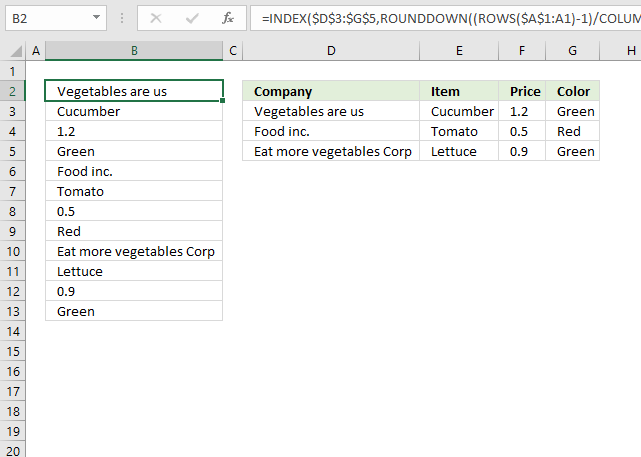The image above demonstrates a formula that returns values from cell range D3:G5 to a single column, in this case, column B.

Formula in cell B2:

=INDEX(\$D\$3:\$G\$5, ROUNDDOWN((ROWS(\$A\$1:A1)-1)/COLUMNS(\$D\$3:\$G\$5),0)+1, MOD(ROWS(\$A\$1:A1)-1, COLUMNS(\$D\$3:\$G\$5))+1)

### 3.1 Explaining formula in cell B2

Step 1 to 3 calculates the first argument (row) in the INDEX function. Step 4 to 6 shows how to calculate the second argument (column) in the INDEX function.

INDEX function syntax: INDEX(array, row_num[column_num])

#### Step 1 - Calculate rows in cell reference

The ROWS function returns the number of rows in a given cell range. Cell reference \$A\$1:A1 grows automatically when the cell is copied to adjacent cells.

The reason it is growing is that cell reference \$A\$1:A1 has an absolute and a relative part, read more here: Absolute and relative cell references

ROWS(\$A\$1:A1)-1

becomes

1-1 and returns 0 (zero).

#### Step 2 - Calculate columns in the cell reference

The COLUMNS function returns the number of columns in a given cell reference.

COLUMNS(\$D\$3:\$G\$5)

returns 4. (D, E, F, and G).

#### Step 3 - Divide first expression with the second expression

(ROWS(\$A\$1:A1)-1)/COLUMNS(\$D\$3:\$G\$5)

becomes

0/4

and returns 0 (zero).

#### Step 3 - Round the result down to the nearest whole number

The ROUNDDOWN function rounds a number down based on the number of digits specified in the second argument.

ROUNDDOWN(numbernum_digits)

ROUNDDOWN((ROWS(\$A\$1:A1)-1)/COLUMNS(\$D\$3:\$G\$5),0)+1

becomes

ROUNDDOWN(0,0)+1

becomes

0 + 1

and returns 1.

#### Step 4 - Calculate rows in the cell reference

The ROWS function returns the number of rows in a given cell range. Cell reference \$A\$1:A1 grows when the cell is copied to cells below or to the right.

ROWS(\$A\$1:A1)-1

becomes

1-1

and returns 0 (zero).

#### Step 5 - Calculate the number of columns in the cell reference

The COLUMNS function returns the number of columns in a given cell reference.

COLUMNS(\$D\$3:\$G\$5)

returns 4 (D, E, F, and G).

#### Step 6 - Calculate reminder if the division

The MOD function returns the remainder after a number is divided by a divisor.

MOD(number, divisor)

MOD(ROWS(\$A\$1:A1)-1,COLUMNS(\$D\$3:\$G\$5))+1

becomes

MOD(1-1,COLUMNS(\$D\$3:\$G\$5))+1

becomes

MOD(1-1,4)+1

becomes

MOD(0,4)+1

becomes

0+1

and returns 1.

#### Step 7 - Get value

The INDEX function gets a value from cell range or array based on a row and column number, the column number is optional if you are working with a cell range that has only one column.

INDEX(\$D\$3:\$G\$5,ROUNDDOWN((ROWS(\$A\$1:A1)-1)/COLUMNS(\$D\$3:\$G\$5),0)+1, MOD(ROWS(\$A\$1:A1)-1,COLUMNS(\$D\$3:\$G\$5))+1)

becomes

INDEX(\$D\$3:\$G\$5, 1, MOD(ROWS(\$A\$1:A1)-1,COLUMNS(\$D\$3:\$G\$5))+1)

becomes

INDEX(\$D\$3:\$G\$5, 1, 1)

and returns "Vegetables are us" in cell B2.